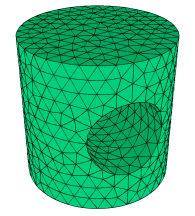﻿ Sample Problems > Usage > 3D_domains > 3d_toggle

# 3d_toggle

Navigation:  Sample Problems > Usage > 3D_domains >

# 3d_toggle{ 3D_TOGGLE.PDE

This problem shows the use of curved extrusion surfaces and VOID layers

to construct a transverse cylindrical hole in an upright cylinder.

 The domain consists of three layers:    1) the cylinder below the hole    2) the hole    3) the cylinder above the hole.  Layer 2 has zero thickness outside the  hole region, and is VOID (excluded  from the mesh) inside the hole.    Click "Controls->Domain Review" to watch  the domain construction process. }   title '3D CYLINDRICAL VOID LAYER TEST'   coordinates    cartesian3   select    errlim = 0.005   variables    u   definitions    k = 0.1    h = 1    L = 1Ro = 1         { the cylinder radius }

Ri = Ro/2       { the hole radius }

{ the base-plane Y-coordinate of the intersection of the hole projection with the

cylinder projection: }

Yc = sqrt(Ro^2-Ri^2)

Z4 = L         { Z-height of the cylinder top }

{ the Z-shape function for the hole top (zero beyond +-Ri): }

Z3 = CYLINDER ((0,1,0), (0,-1,0), Ri)

{ the Z-shape function for the hole bottom (zero beyond +-Ri): }

Z2 = -Z3

Z1 = -L         { Z-height of the cylinder bottom }

equations

U: div(K*grad(u)) + h = 0       { a heat equation for demonstration purposes }

extrusion z=Z1,Z2,Z3,Z4     { short-form specification of the extrusion surfaces }

boundaries

Region 1       { this region is the projection of the outer cylinder shape }

start(Ro,0)

value(u)=0               { Force U=0 on perimeter }

arc(center=0,0) angle=360 to close

limited region 2       { this region is the projection of the transverse hole }

layer 2 void         { the region exists only in layer 2.  Its bounding surfaces

merge beyond the edges of the hole  }

start(Ri,Yc) arc(center=0,0) to (-Ri,Yc)

line to (-Ri,-Yc)

arc(center=0,0) to (Ri,-Yc)

line to close

monitors

grid(x,y,z)

elevation(u) from (-Ro,0,0) to (Ro,0,0)

contour(u) on z=0

contour(u) on y=0

plots

grid(x,y,z)

elevation(u) from (-Ro,0,0) to (Ro,0,0)

contour(u) on z=0

contour(u) on y=0

end